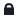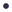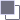###GUNS OF TEH AWESOME

0 Members and 1 Guest are viewing this topic.ZekeySpaceyLizard« Reply #60 on: November 28, 2008 03:24 PM »
Hello I'm ZekeySpaceyLizard
Much like F00d and Raptor I too have some very important things to get off my chest on a public internet forum so here they go:

Animation is like most introductory PDE courses spend a great deal of time investigating solutions  using separation of variables, a powerful method that is good to know for studies in theoretical physics is the method of eigenfunction expansion. This method is useful because in many cases, equations cannot be solved using separation of variables. To understand the method, in what follows you can think in terms of expanding the function which solves the equation in a Fourier series. We then solve mode by mode-turning a single partial differential equation into a set of elementary ordinary differential equations that can be solved by inspection. Rather than trying to explain the techniques in words or with difficult to understand abstract theorems, letâ€™s demonstrate how to use the method with two concrete examples.In our first attempt to tackle the method of eigenfunction expansion, we will consider a standard example from quantum mechanics, a particle trapped in an infinite square well of width a. Inside the well, the potential is zero and therefore the Schrodinger equation is written as
For this problem we will take . The particle cannot be found outside the boundaries, therefore the boundary conditions for the problem areAs can be seen in any standard quantum mechanics text like Griffiths, this problem is easily solved using separation of variables. While that elementary option is available in this case, we will use the method of eigenfunction expansion so that we can demonstrate the method using a simple equation.
The first step is to identify a differential operator L that can be used to solve an eigenvalue equation. In problems that involve space and time, its  a good bet to choose spatial derivative operators as L. In this case the choice is immediately obvious. We let f.

But see the thing about newgrounds is We denote the eigenfunctions of L by  and the eigenvalues by . The eigenvalue equation is then written as For convenience, we set  (the reader can later verify that this would follow automatically). The differential equation to be solved is then written as This part of the solution will be familiar to those who have had quantum mechanics. Basically, we are solving the time-independent Schrodinger equation. Students will no doubt recognize the above equation as the harmonic oscillator equation with solutionsThe boundary conditions in the problem tell us that. This applies to the eigenfunctions as well. Therefore, we find that Now since , this means that . Next we apply the second boundary condition, . This gives In quantum theory, the constants can be found using normalization. However that isnâ€™t necessary to understand the solution method being explored here, so we will ignore it from here on out. The reader familiar with quantum theory will also note that the eigenvalues  are related to the energy.

But Tom Fulp well...

Tom Fulp often etâ€™s equate both terms from the left hand side and the right hand side. The Schrodinger equation becomes We now have two series expansions using the same eigenfunctions that are equal, therefore each individual term in the series on the left must equal the corresponding term on the right. This means that the coefficient functions satisfy the following ordinary differential equation

Normally, the constant of integration would be determined using initial conditions stated in the problem. In this case once again normalization determines the constants in this problem. We will ignore what those values are and simply write the solution, which isWe will follow the same procedure used in the first example. First we identify a differential operator L and eigenfunctions  such that
The are numbers called eigenvalues whose form we will determine. Once we have the eigenfunctions, we write f in a series expansion then plug it into the differential equation, and solve mode by mode for the coefficient functions .  Letâ€™s see how to apply the method to the equation we are trying to solve in this case. We have . Looking at the right hand side, we see that the differential operator (acting with respect to x) iswith      . Now, since we are only dealing with a function of a single variable x, we can write the equation in terms of ordinary derivatives. Moving all terms to the right hand side, we obtain the familiar simple harmonic oscillator equation From our knowledge of the sin function, we know that this can only be true if the argument is an integer. This is why we have labeled the eigenvalues with the subscript as . Letâ€™s call that integer n, and so we have Putting this together with , we conclude that the eigenfunctions of the given differential operator and stated boundary conditions are  with eigenvalues
The next step is to write  in an eigenfunction expansion, absorbing the time dependence into the coefficients of the expansion. With , we can write We changed to ordinary derivatives because the coefficient functions are functions of t only. Next we consider the second derivative with respect to x in the first term on the right hand side. Using the same procedure, we have The remaining term on the right hand side, , is the forcing function which we denote . Generally we can expand the forcing function in an eigenfunction expansion, using the same eigenfunctions but different coefficients. That is So we see that  and all other terms are zero. This greatly simplifies the problem. Now letâ€™s put everything together. Using the eigenfunction expansions of each term, we have rewritten the PDE in the following way We need one more piece of the puzzle-the initial conditions. Looking at the problem statement, we were told that . Comparing this with , we conclude that the initial conditions applied to the coefficient functions of the expansion gives us . Since setting in , we conclude that  Also remember that the only nonzero forcing term is . So this leaves two equations to solve. The first is Foamy the Squirrel

The internet sometimes runs on a loop where The last piece of the puzzle is to solve the equation involving the forcing term, where . In this case,  so we need to solve The -16 comes from the expansion, where we hadThe homogeneous equation is With solution , C being the constant of integration. Since the inhomogeneous term is a constant, we guess that the particular solution is also a constant, call it A. So we have tells us that it must be the case that , and so we take . To write down the final solution, we just write down a summation of the non-zero coefficient functions  multiplied by . And so we have Notice it satisfies the initial condition  (set t = 0). What about long term behavior? This is called steady state behavior. We take the limit  and find that the steady state solution is fucking a penguin up its tight asshole

and thats all i have to say on the matter
« Last Edit: November 28, 2008 03:26 PM by Zekey »Quote from: Kirbopher
The section where she was retrieving the eyes of the three ghost kids seemed ridiculously Zelda-like.
Quote from: Kirbopher
Never once said "the movie reminded me of Zelda" nor am I even talking about the END
Quote from: Kirbopher
Obviously, but again, that wasn't even what I was saying, nor was I even implying it to be something negative.

FUCK YOU FUCK YOU FUCK YOU
<rtil> wow that dick's got some torque